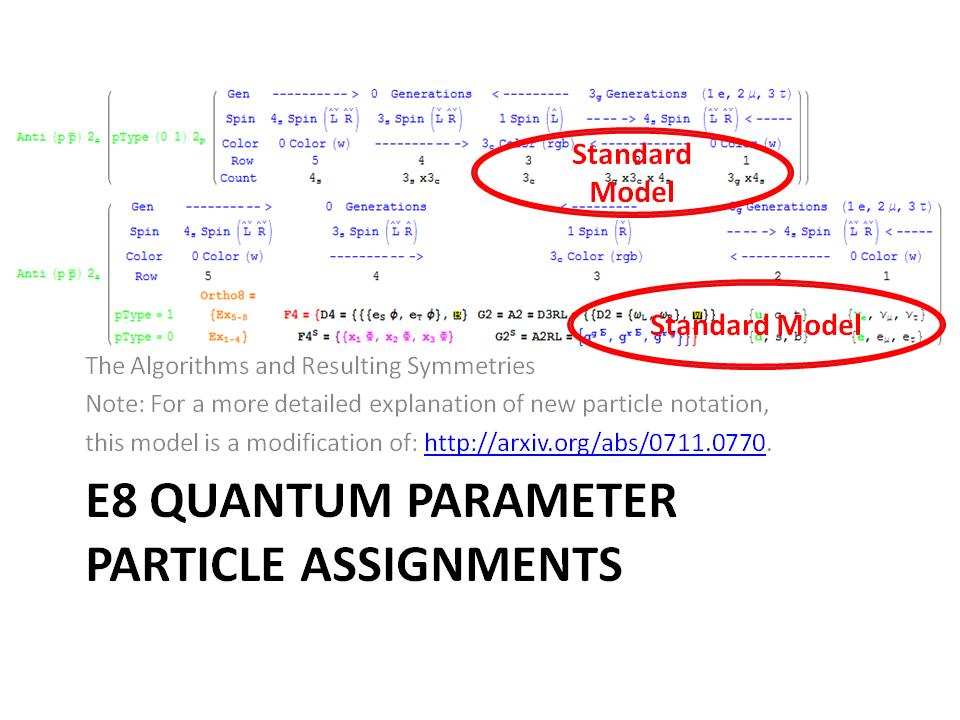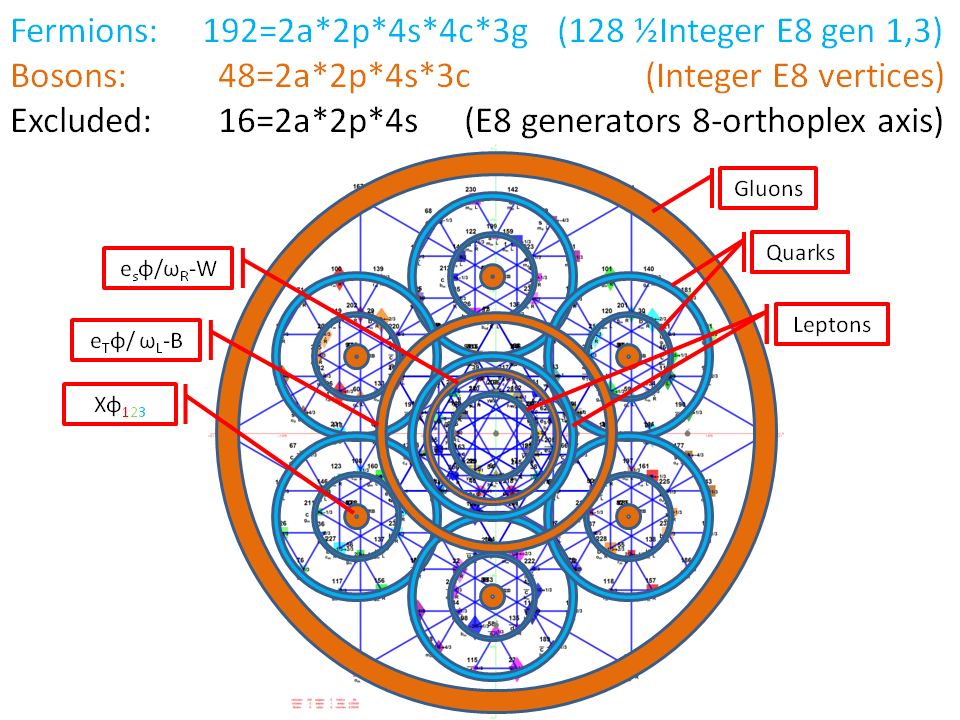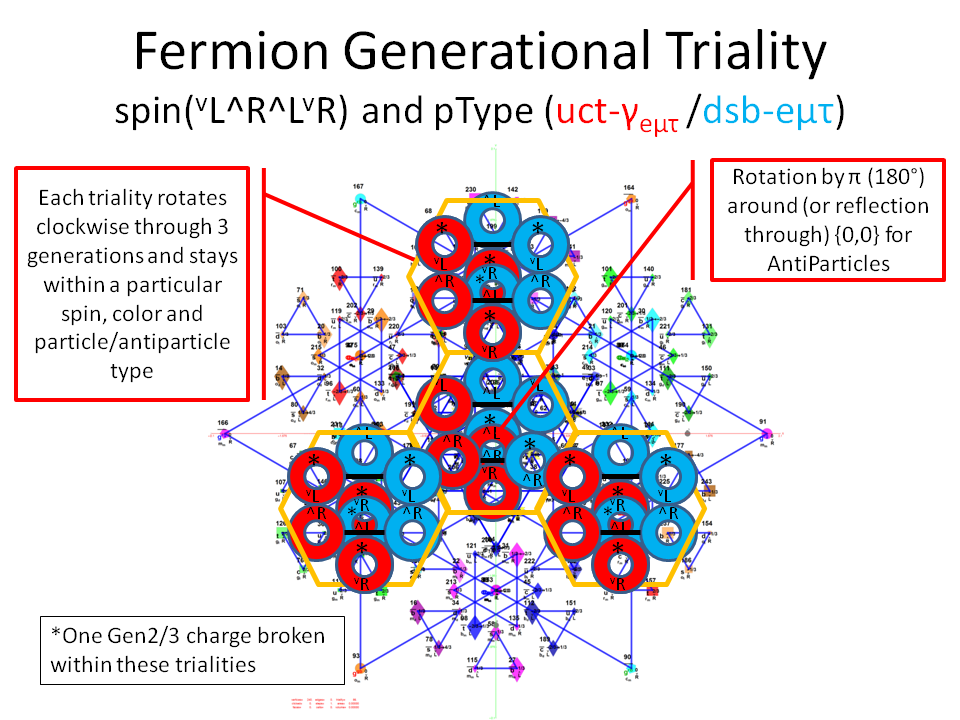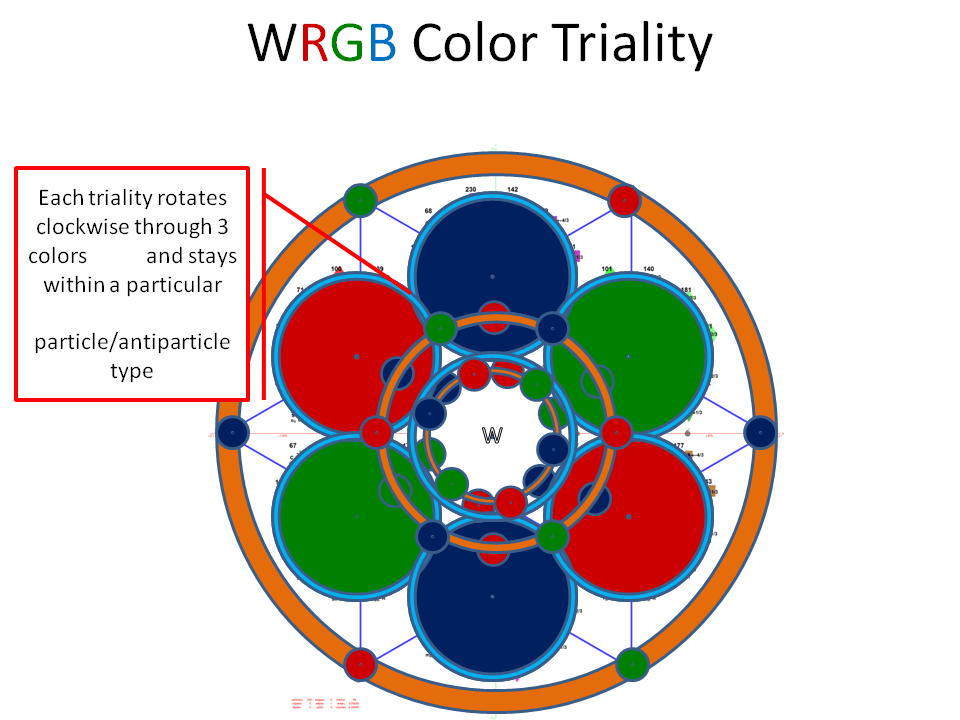# Working with E8+++

I wanted to confirm some work being done with E11 (or more specifically the Extended E8+++), so I used my “VisibLie” notebook (which includes the “SuperLie” package for analyzing Lie Algebras) to get the following information:

I first created the Dynkin diagram which produces the Cartan Matrix: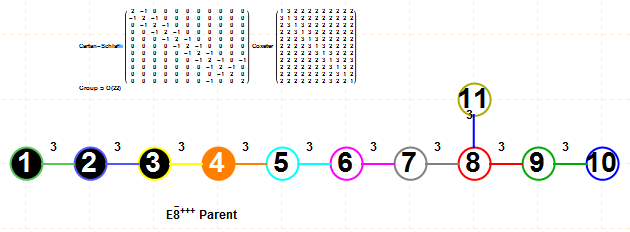This allows the evaluation of the EigenSystem of the Cartan matrix as follows: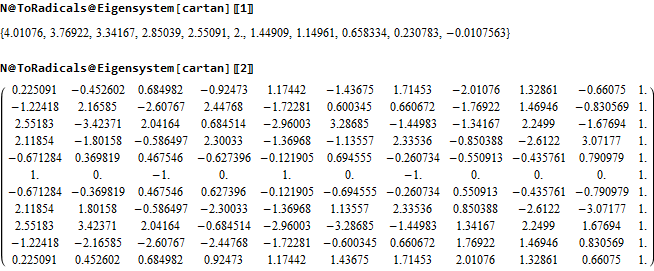As well as using the SuperLie package to evaluate the Positive Roots, Weights and Heights of the E8+++ (The first 80 are shown. For the full list in .pdf click here or click on the image below):The 4870 positive roots up to height 47 generate a Hasse diagram as follows (with 3 sections zoomed in):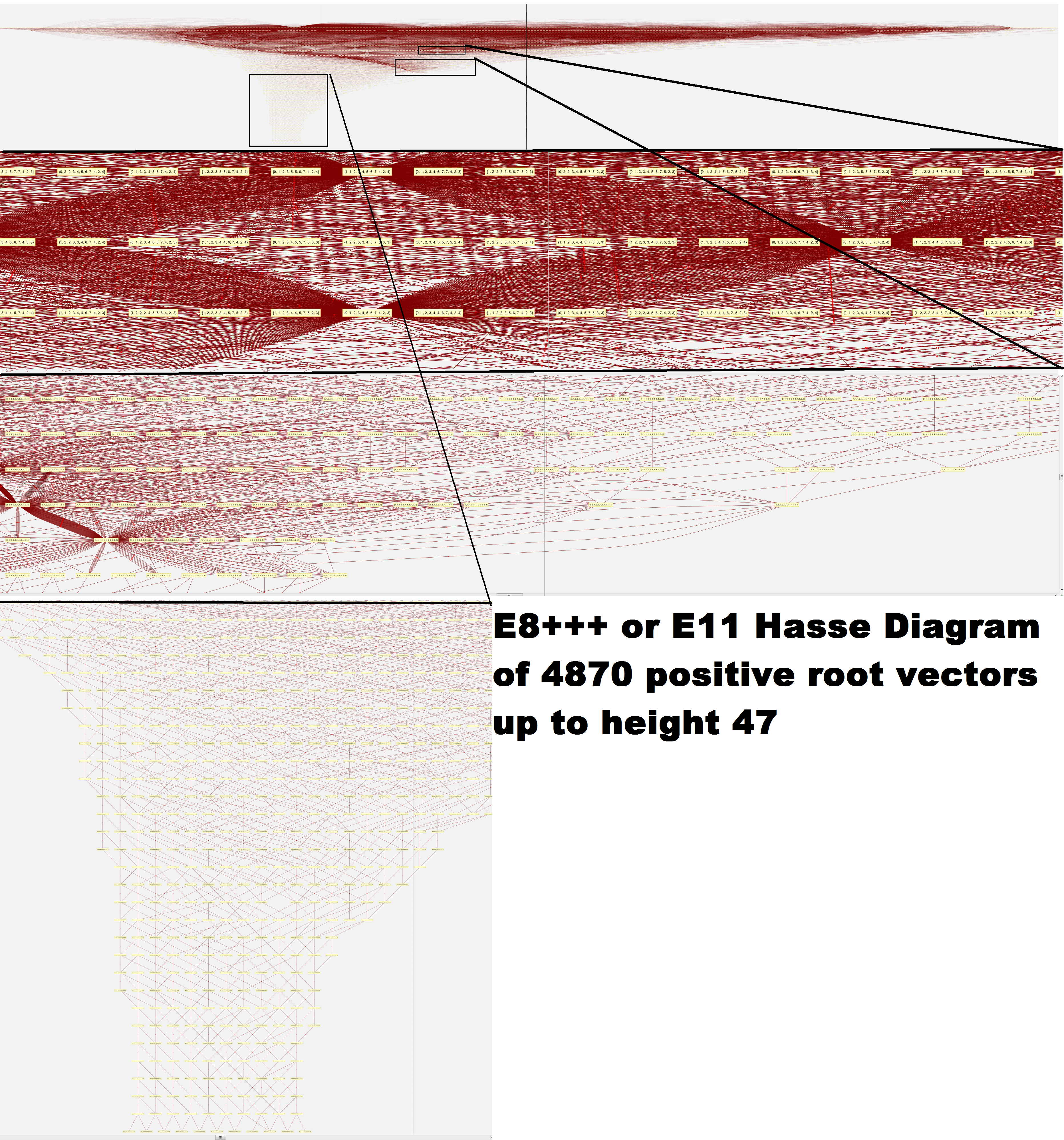I am working on replication of the Petrie projection by A.G. Lisi based on his prescription using his basis vectors for 2D projection and a Simple Roots Matrix: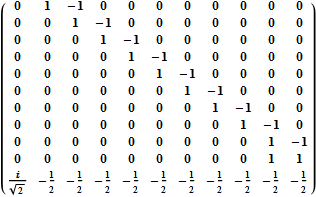Adjusting this E11 Simple Roots Matrix (srmE11) to match the Dynkin diagram above and then verifying it creates the same Cartan matrix, we have: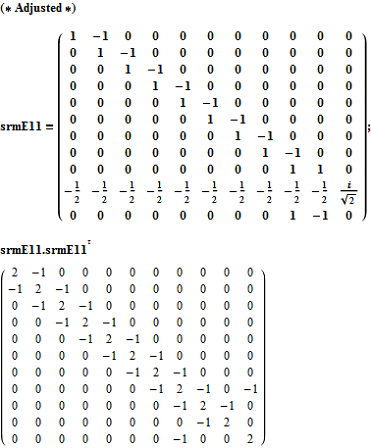To get the 9740 E8+++ vertices, we simply use the dot product of the transpose of srmE11 against the positive and negative root vectors (first 50 shown):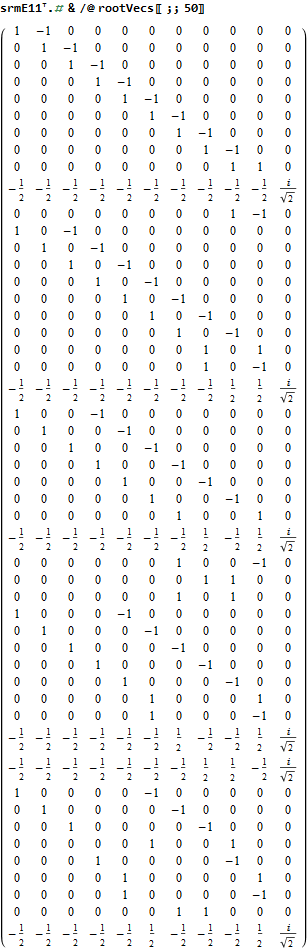A first cut at plotting these involves projecting them to a 2D using the dot product of the 2 basis vectors provided, which gives the following ListPlot: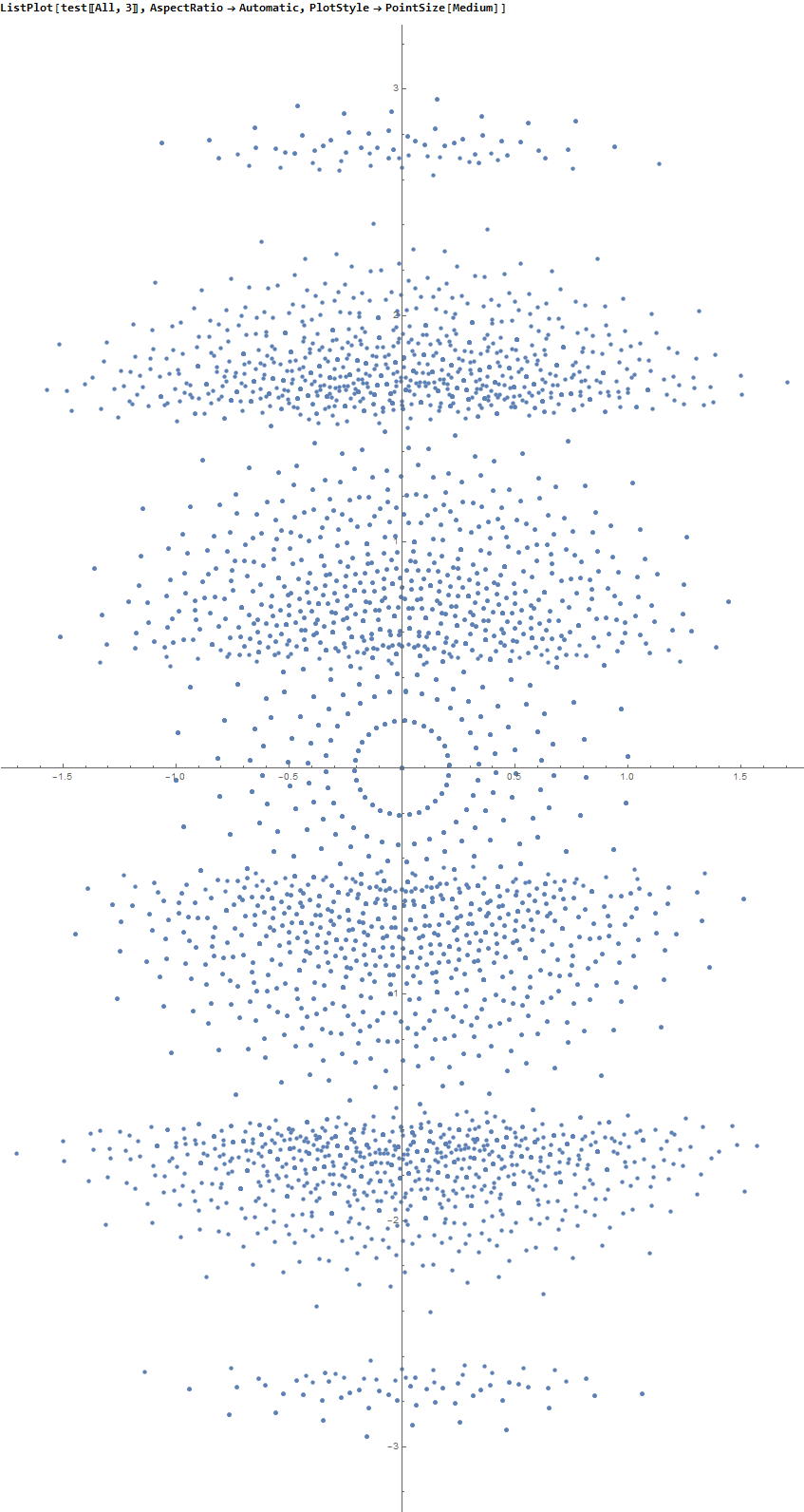The limited results from SuperLie are consistent with the image given by Lisi on the Bee’s BackReaction blog, after eliminating the vertical “levels” in my raw plot.

Email me if you want more detail, see errors, or would like to help.

Greg
JGMoxness@theoryofeverything.org

# 3D Printed E7 1_32 Polytope Projected to the E6/F4 Coxeter Plane

The 3D model of the polytope: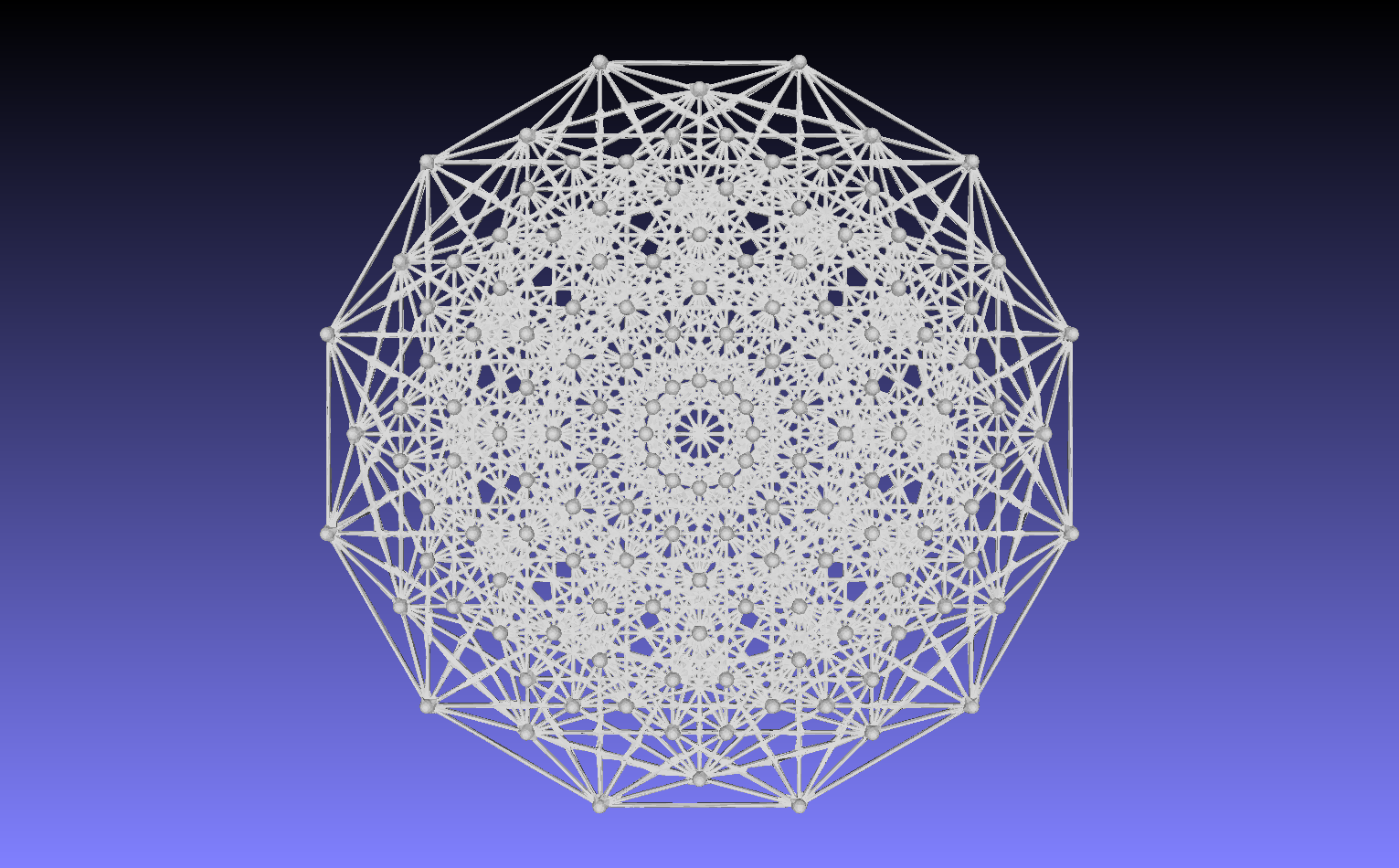3D printed in raw bronze:
Front: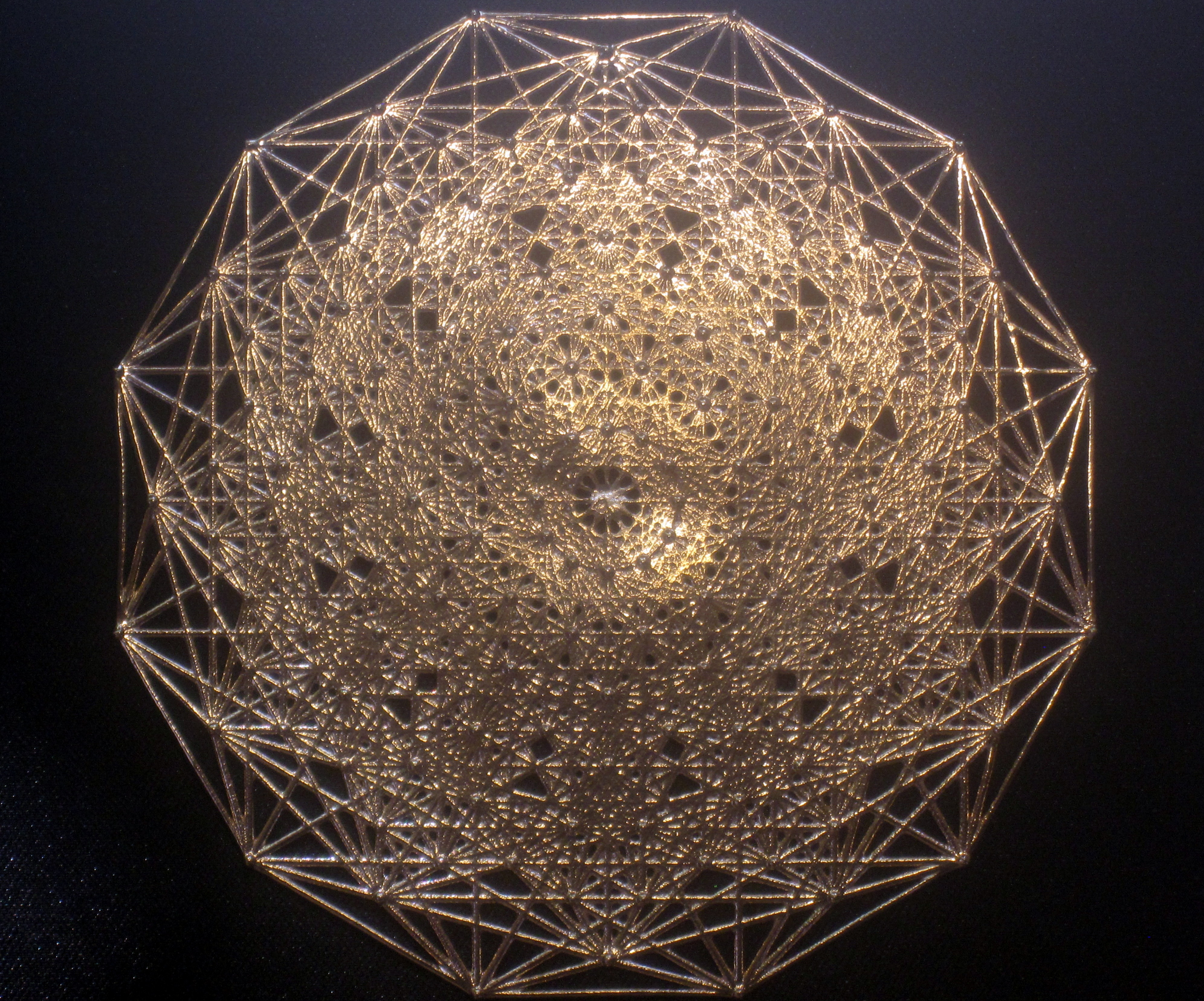Back:Here it is in a 3 1/8″x3 1/8″ laser etched optical crystal.# Star of David Projection Basis for E8, E7 and E6

For a full discussion of the particle assignment symmetries involved, see http://vixra.org/pdf/1503.0190v1.pdf

E8 as 240 split real even vertices with  vertex color, size, shape, and labels from a modified A.G. Lisi particle assignment algorithm. The vertex number is the position in the Pascal Triangle (Clifford Algebra) representation of E8. The blue lines forming equilateral triangles represent the  10 Bosonic (or Color) Triality (T2) and 76 Fermionic (T4) rotations (using the 8×8 matrices shown below) that transform each member of the triangle into the next member.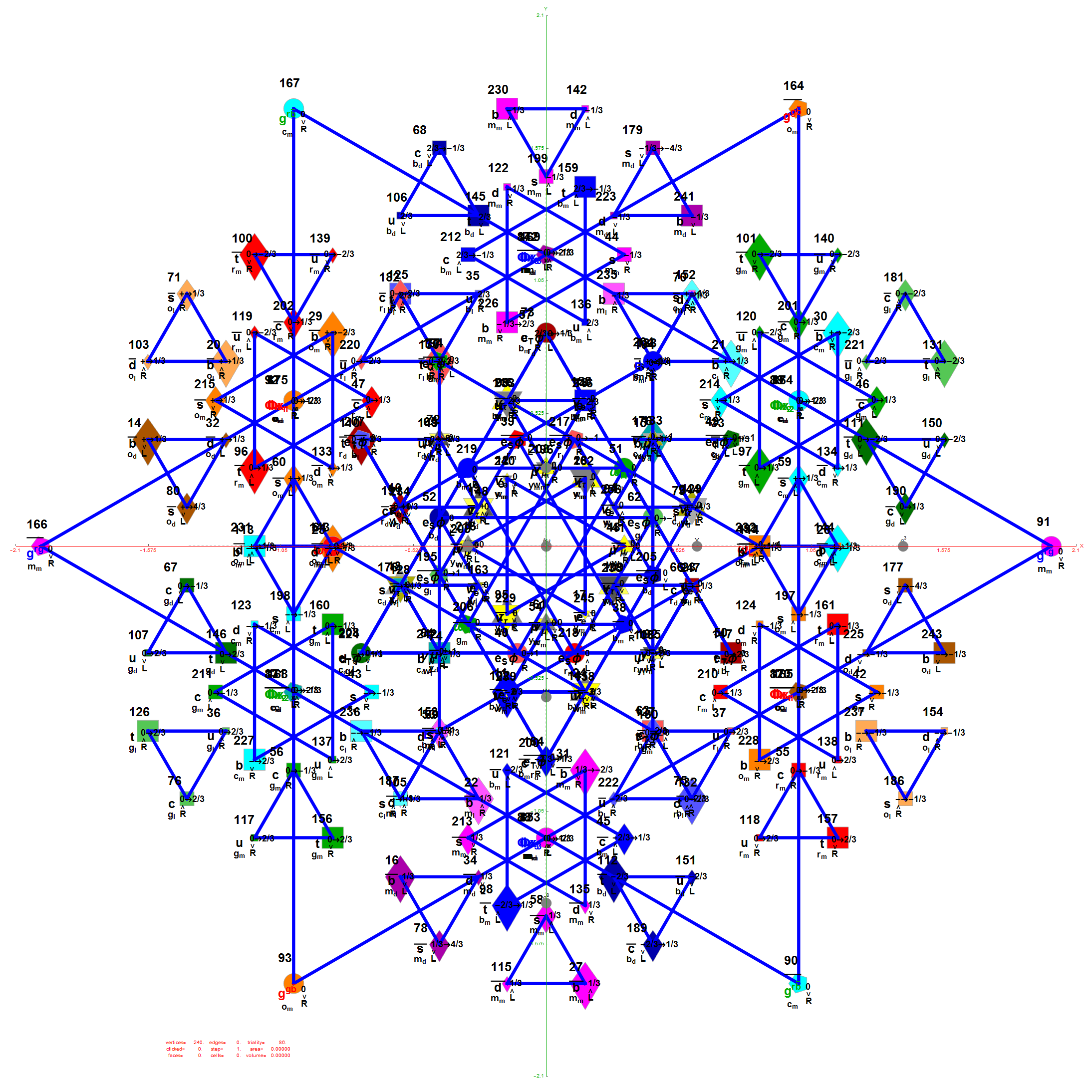E7 as derived from E8 above by taking the vertices of E8 with the last two columns being equal.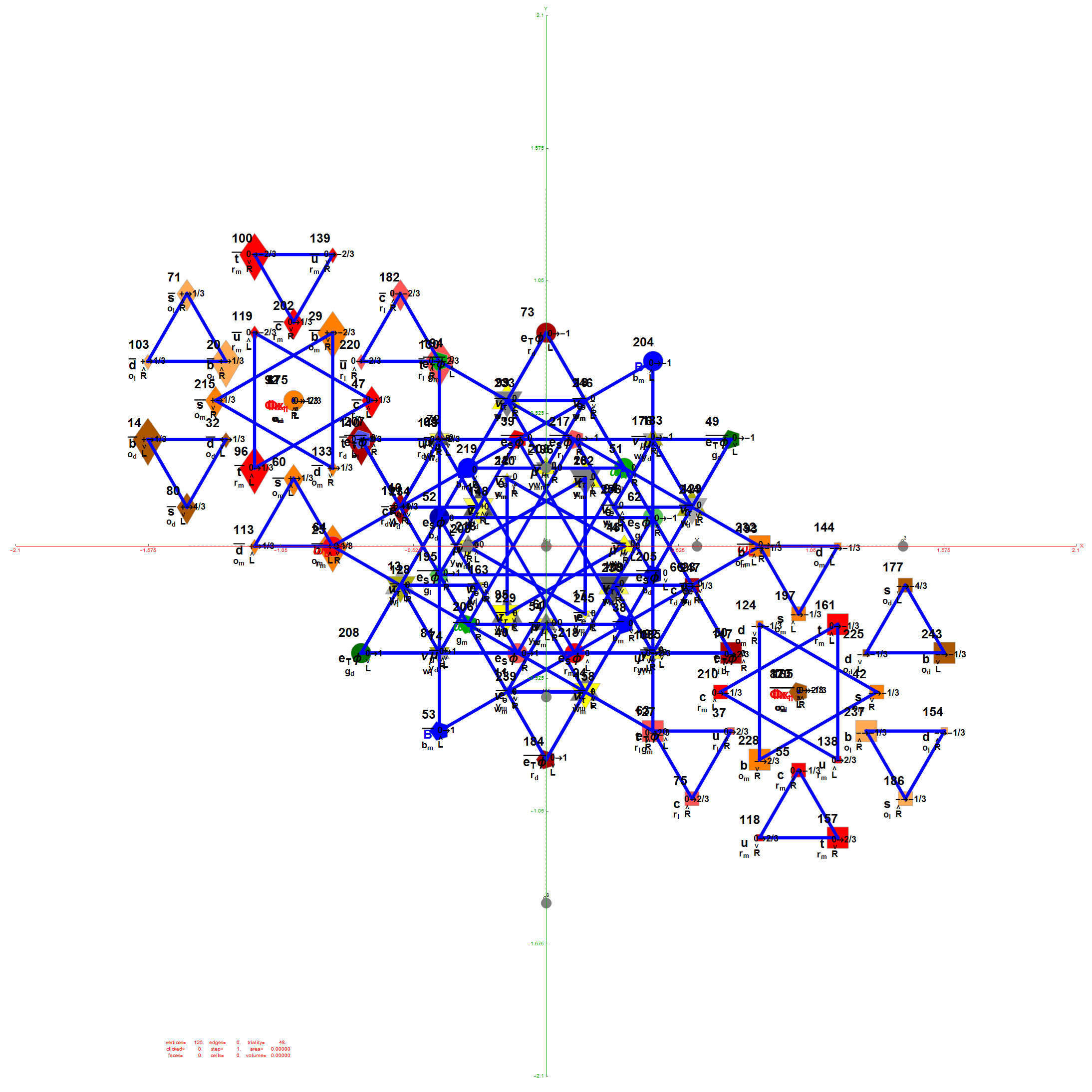E6 as derived from E8 above by taking the vertices of E8 with the last three columns being equal.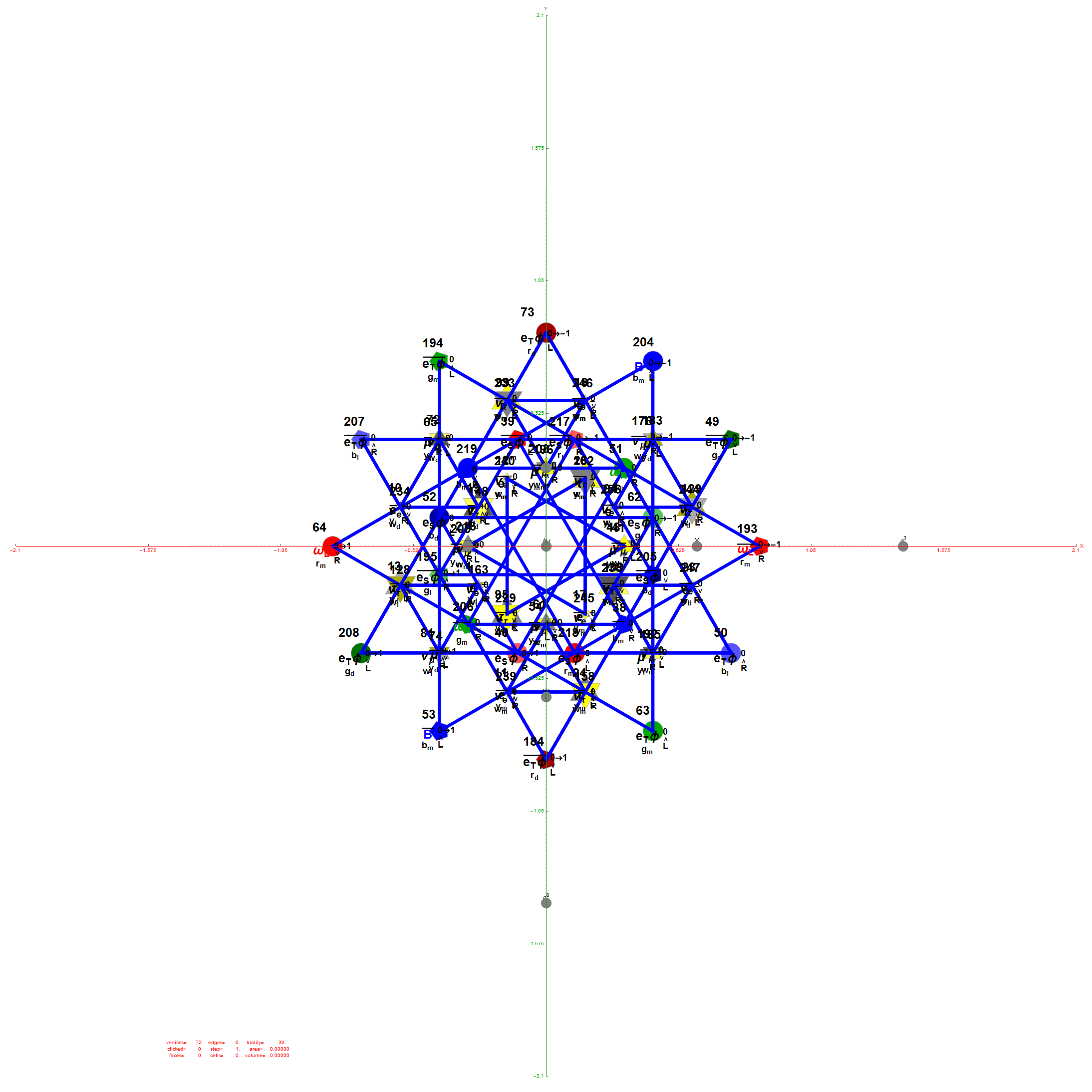The 48 vertices of F4 are made up of the 112 (D8) +/-1 integer pair vertices that are assigned to bosonic particles, which includes 22 trialities (10 from T2 and 12 from T4). The other 64 of 112  D8  vertices are assigned to the 2nd generation of Fermions. F4 is shown below with its sub-group G2 assigned to the gluons in the larger triality triangles.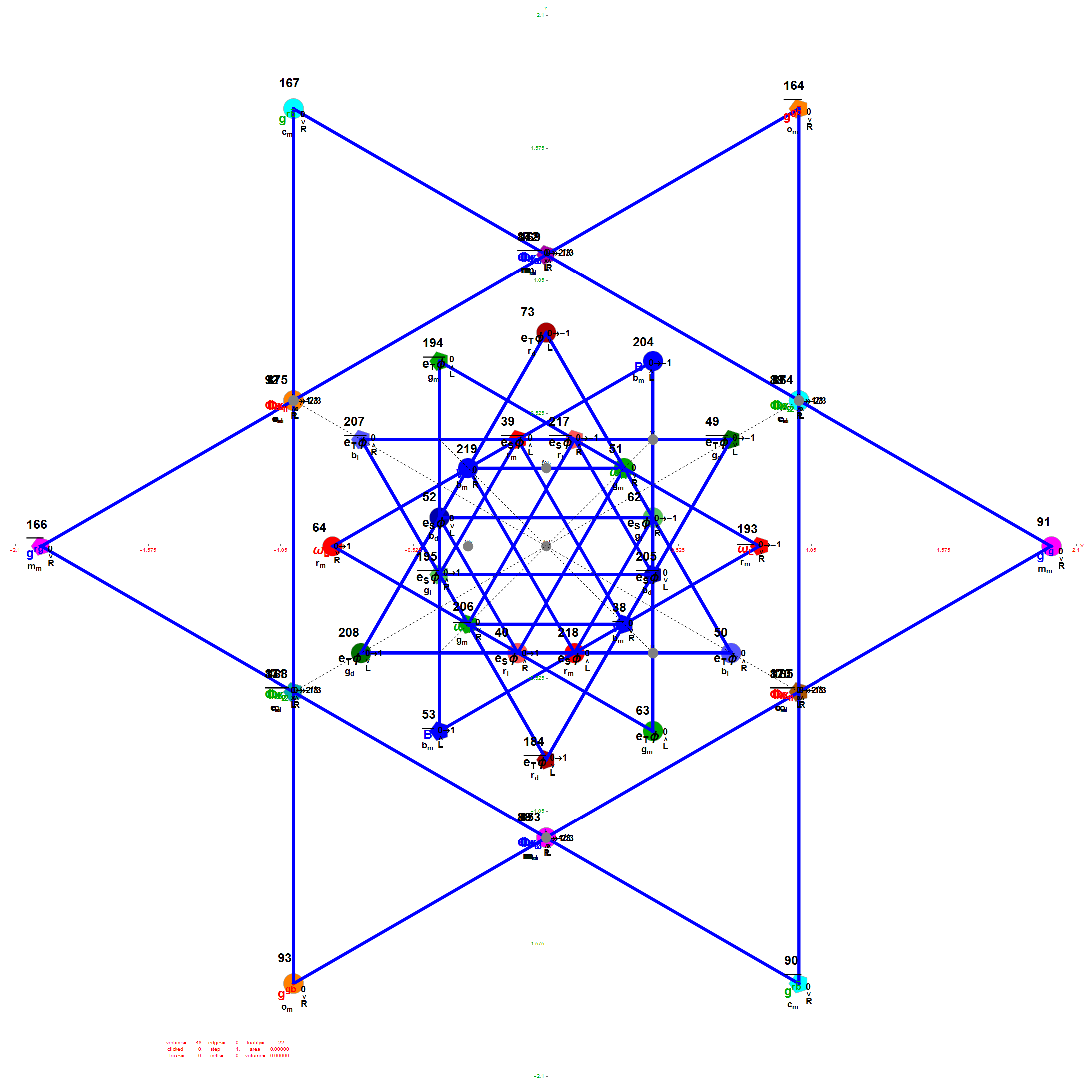Projection Basis for X and Y are H (first row) and V (second row) in the matrix below respectively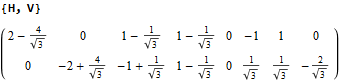The Triality Matrices are: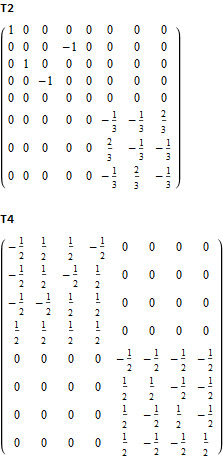The 10 Bosonic (Color) Triality Rotation Matrix (T2) equilateral triangles exclusively affect the particles that are derived from F4.

T2 rotates through the bosonic colors indicated by the last 3 columns of the E8 vertices appropriately labeled (r,g,b).The 76 Fermionic (Generation) Triality Rotation Matrix (T4) equilateral triangles. There are 64 fermion particle trialities that are derived from the 128 (BC8) half integer vertex assignments plus the 64 fermions assigned from the 112 (D8) +/-1 integer pair vertices. There is always one integer (2nd Generation) and two 1/2 integer vertices (1st and 3rd Generation) in every fermionic triality.

That is, T4 rotates through the fermionic generations.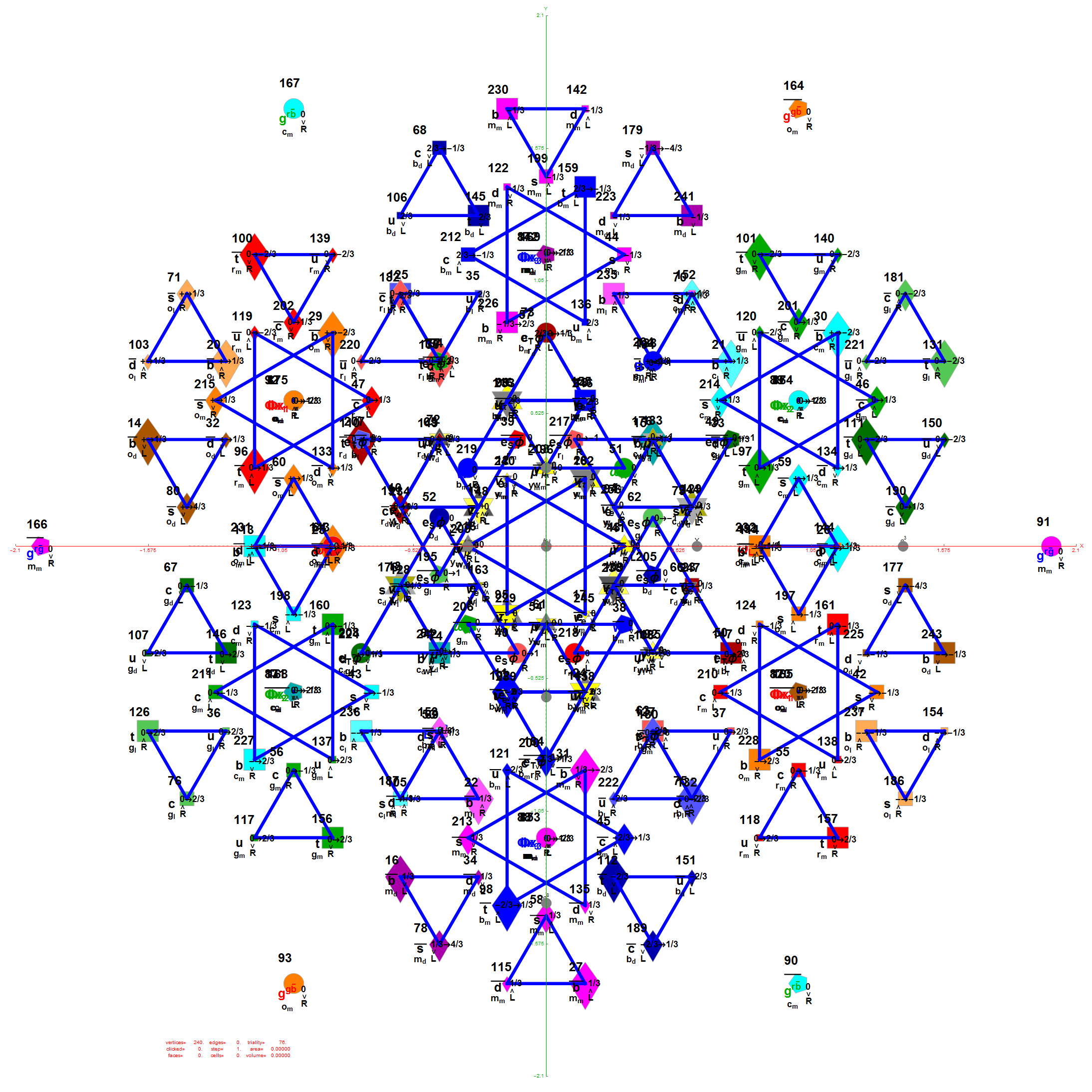There are 12 trialities from these 76 that are associated with the inner E6 & F4 Lie Group vertices assigned to bosons, shown below with only the 112 (D8) +/-1 integer pair unlabeled vertices: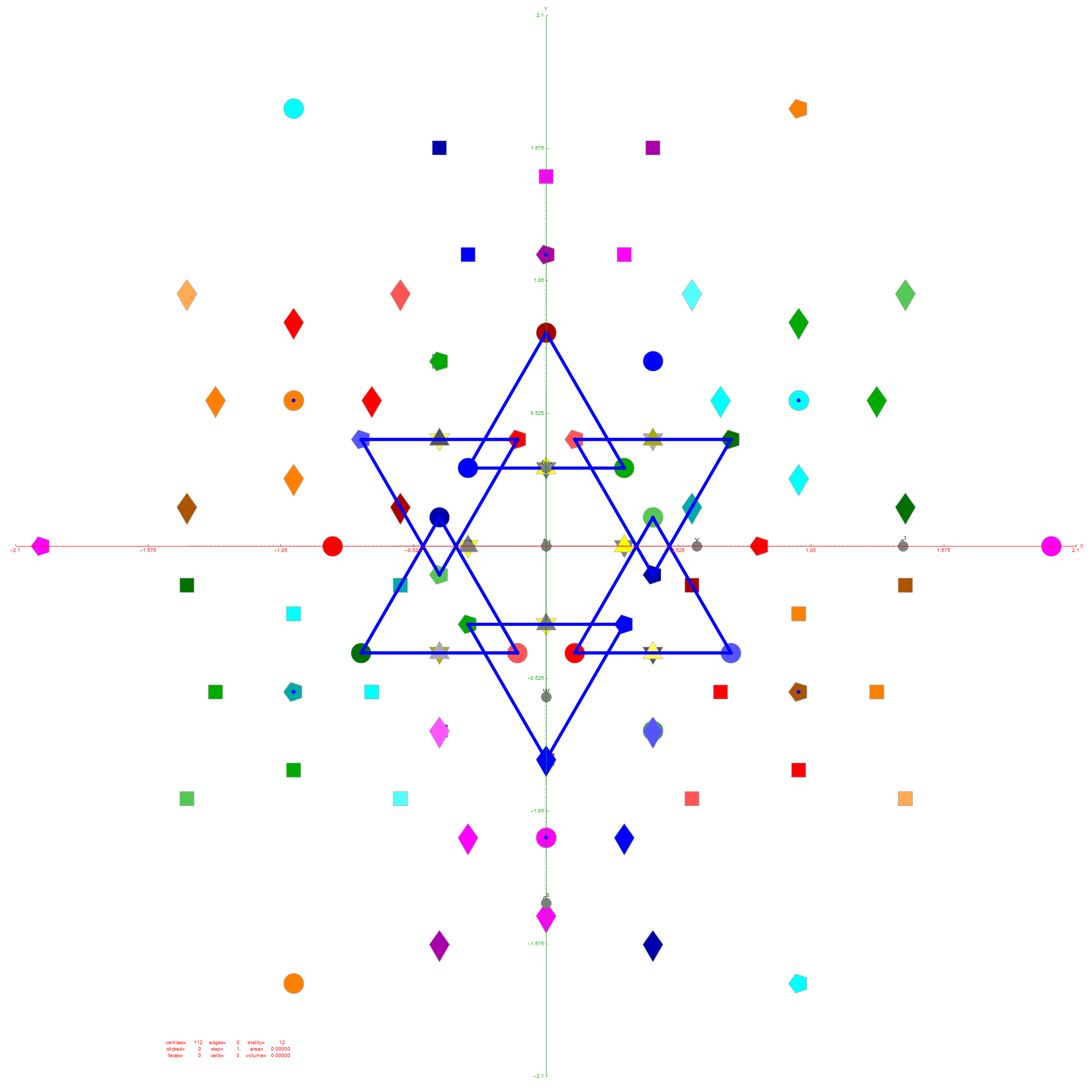Of course, there are a number of overlapping vertices in this projection stemming from the 112 (D8) +/-1 Bosonic sector. The following image colors the vertices by overlap count, with 120 Yellow with no overlaps, 24 Cyan with 2 overlaps (48), and 24 Cyan with 3 overlaps (72).

Notice the 8 projection basis vectors with dark gray circles. Of course, these projection basis vectors are the 8 “generator vertices” of E8 vertices with permutations of {+1,0,0,0,0,0,0,0} giving it the full 240+8=248 dimension count. Add to that the -1 “anti-generators” being the opposite end of the projection basis vectors and you get the full 256 vertices representing the full Clifford Algebra on the 9th row of the Pascal Triangle.There are 6720 edges in E8 with an 8D length Sqrt.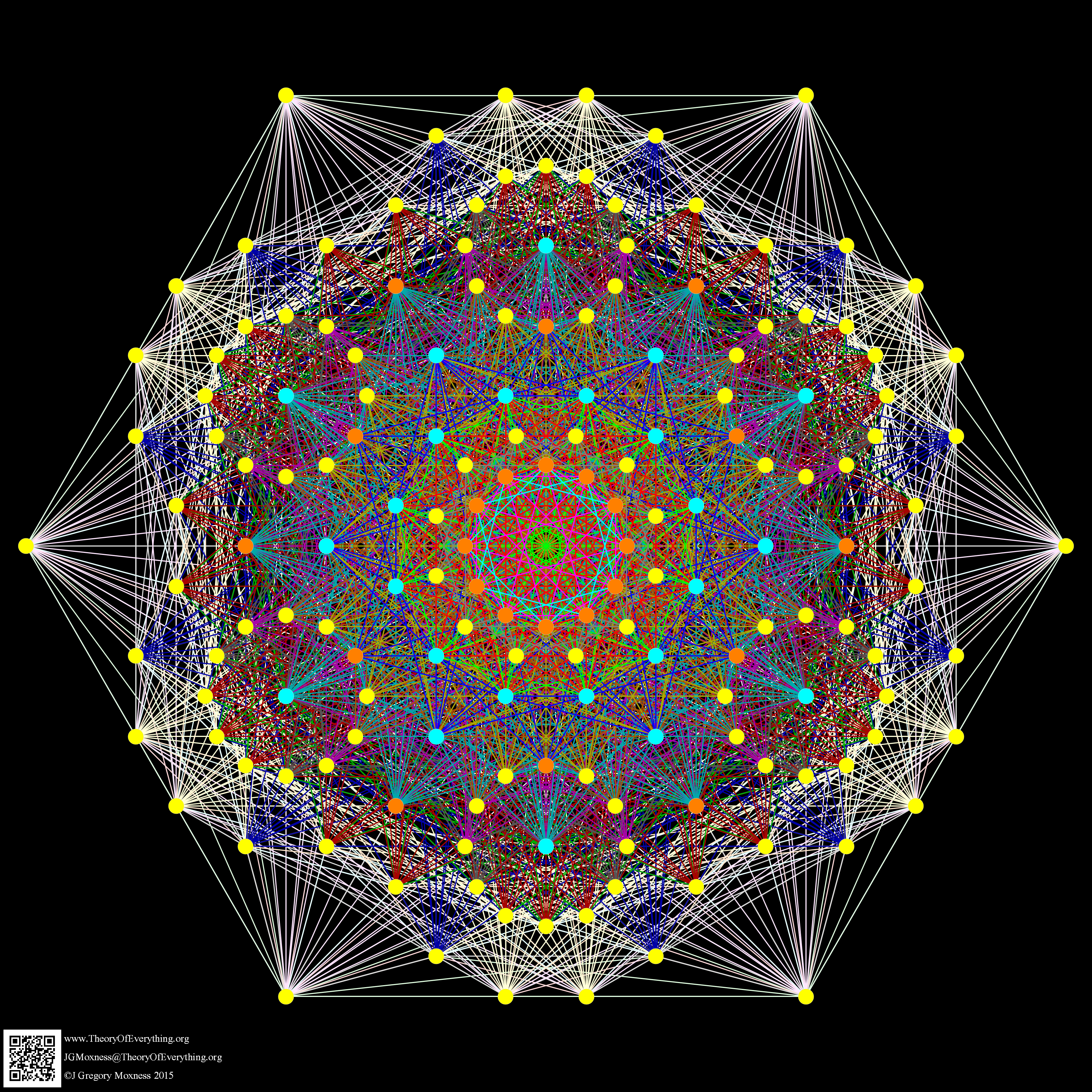Just for fun, I introduce to you the 6720 rectified E8 vertices taken from the midpoint of each edge and using the same projection with coloring of the spheres based on overlap count. Enjoy!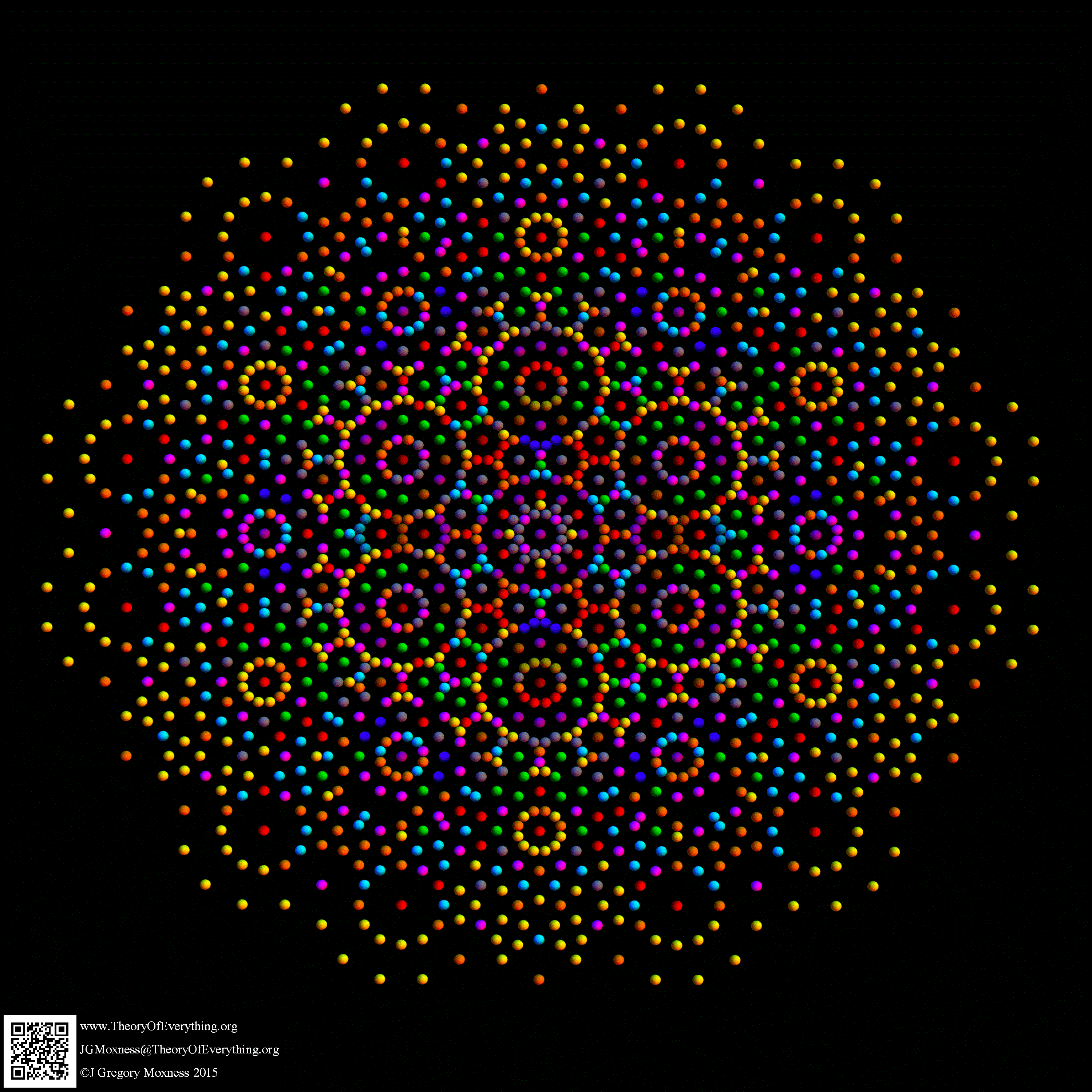Here are the G2 gluons connected by their trialities shown in a 3D concentric hull projection of E8 using the E8 to H4 folding matrix basis vectors. This is the 4th hull, which is the outer hull of the inner H4 600 cell (an icosadodecahedron). For more on E8 hulls, see this post.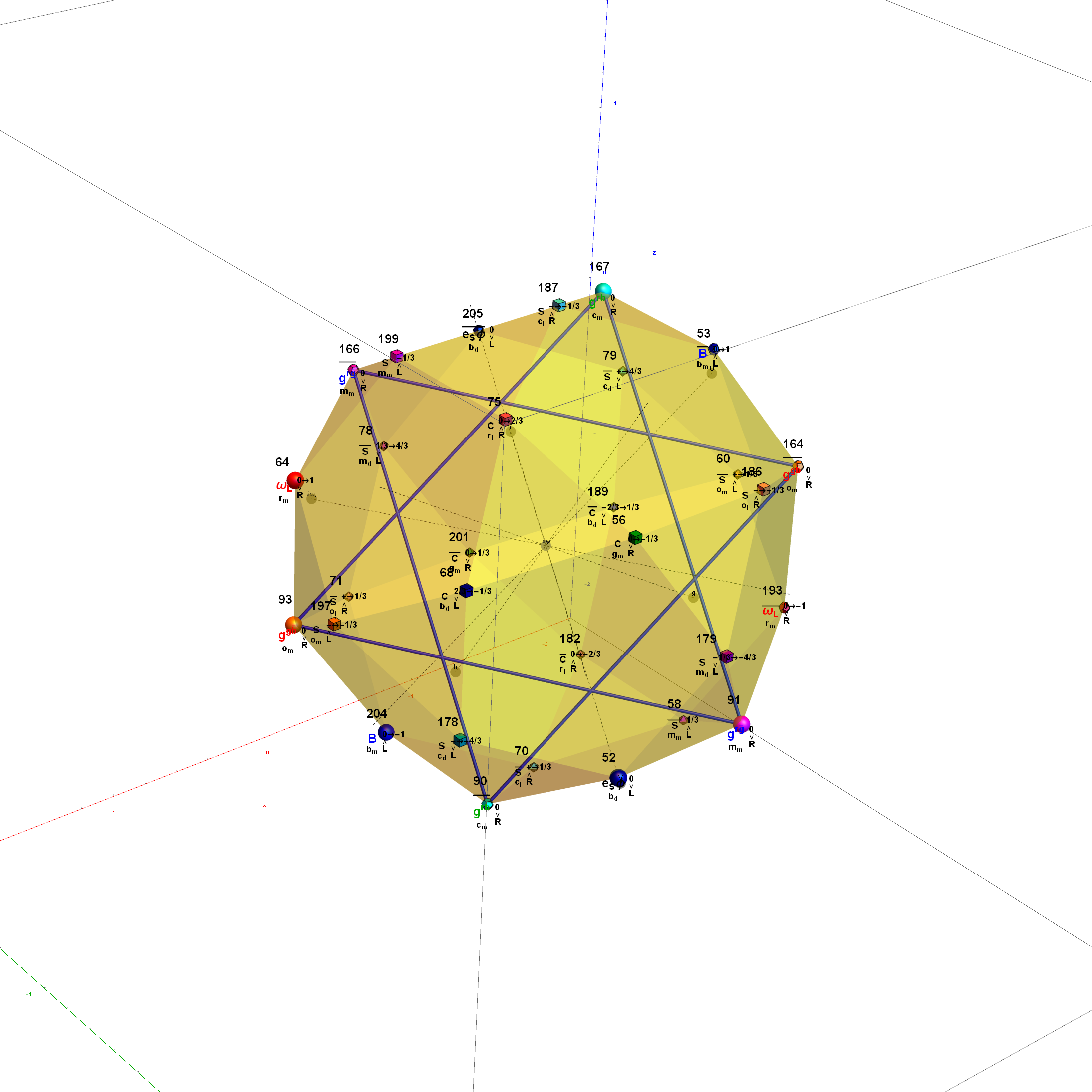The full F4 group with 10 T2 and 12 T4 trialities affecting the bosons is contained in the outer icosadodecahedron (1st hull) combined with the 3rd (quad icosahedral) and 4th icosadodecahedron hulls.Below is the full E8 with all trialities shown in 3D concentric hull projection.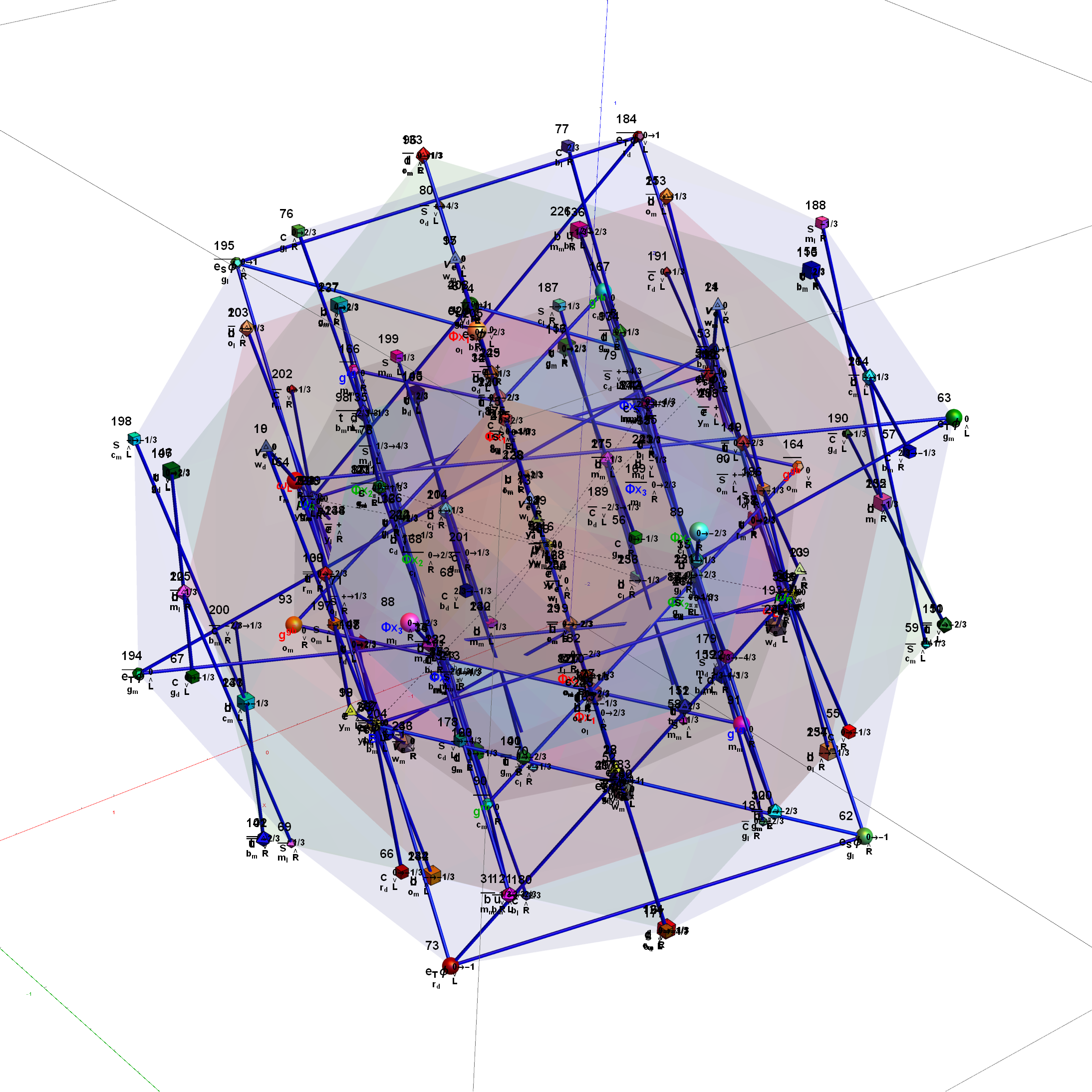#### Wow – that is nice! Remember, you heard it here first!

BTW – if you find this information useful, or provide any portion of it to others, PLEASE make sure you cite this post. If you feel a blog post citation would not be an acceptable form for academic research papers, I would be glad to clean it up and put it into LaTex format in order to provide it to arXiv (with your academic sponsorship) or Vixra. Just send me a note at:  jgmoxness@theoryofeverthing.org.

# Comparing nearest edges between Split Real Even (SRE) E8

I have been intrigued with Richter’s arXiv 0704.3091 “Triacontagonal Coordinates for the E8 root system” paper.

Comparing nearest edges between Split Real Even (SRE) E8 and Richter’s Complex 4D Golden Ratio Petrie Projection Model:

Please note, the vertex numbers are reordered from Richter’s paper in order to be consistent with ArXiv quant-ph 1502.04350 “Parity Proofs of Kochen-Specker theorem based on the Lie Algebra E8” by Aravind and Waegell. This paper uses the beautiful symmetries of E8 as a basis for proof sets related to the Bell inequalities of quantum mechanics. Below is a graphic showing that vertex number ordering: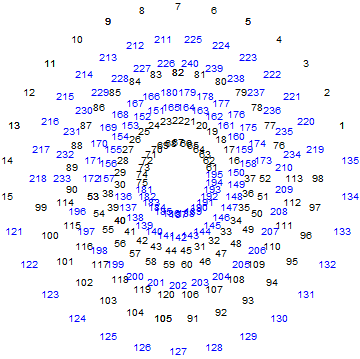Richter’s model with 74 nearest edges per vertex (Complex 4D Norm’d length Sqrt, with 8880 total):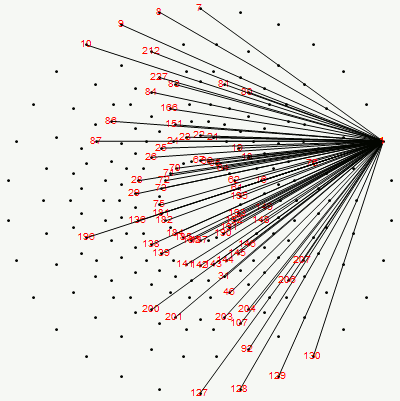Richter’s model with only 26 nearest edges per vertex (Complex 4D Norm’d length 1, 3120 total):The above unity length edge pattern has is more consistent with the SRE E8 below.

The SRE E8 Petrie Projection (with 56 Norm’d edge length Sqrt for each vertex, 6720 total). This is equivalent to folding E8 to H4 with an 8×8 rotation matrix which creates a 4D-left H4+H4*Phi and a 4D-right H4+H4*Phi.

Please note, the vertex number is that of the E8 vertices rotated (or “folded”) to H4 in 2D Petrie projection. The vertex numbers are in canonical binary order from E8’s 1:1 correspondence with the 9th row of the Pascal Triangle (eliminating the 16 generator/anti-generator vertices of E8 found in the 2nd and 8th column of the Pascal Triangle). The graphic below shows the vertex number ordering: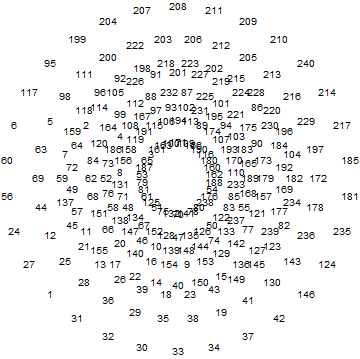Here we take the 4D-left half and project to two concentric rings of H4 and H4 Phi (Golden Ratio) with 56 nearest edges per vertex (Norm’d length of unity or Phi for each vertex):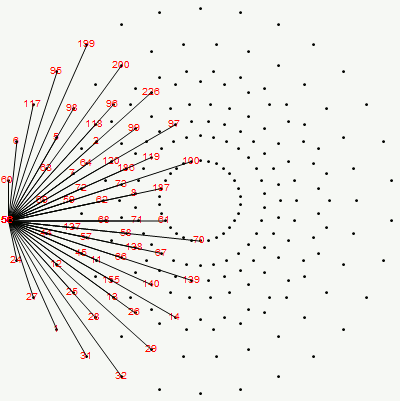The animation frames are sorted by the ArcTan[y/x] of the vertex position in each ring (sorted H4*Phi outer to inner, then H4 outer to inner).

BTW – if you find this information useful, or provide any portion of it to others, PLEASE make sure you cite this post. If you feel a blog post citation would not be an acceptable form for academic research papers, I would be glad to clean it up and put it into LaTex format in order to provide it to arXiv (with your academic sponsorship) or Vixra. Just send me a note at:  jgmoxness@theoryofeverthing.org.

# E8 in E6 Petrie Projection

An article (interview) with John Baez used an E8 projection which I introduced to Wikipedia in Feb of 2010 here. Technically, it is E8 projected to the E6 Coxeter plane.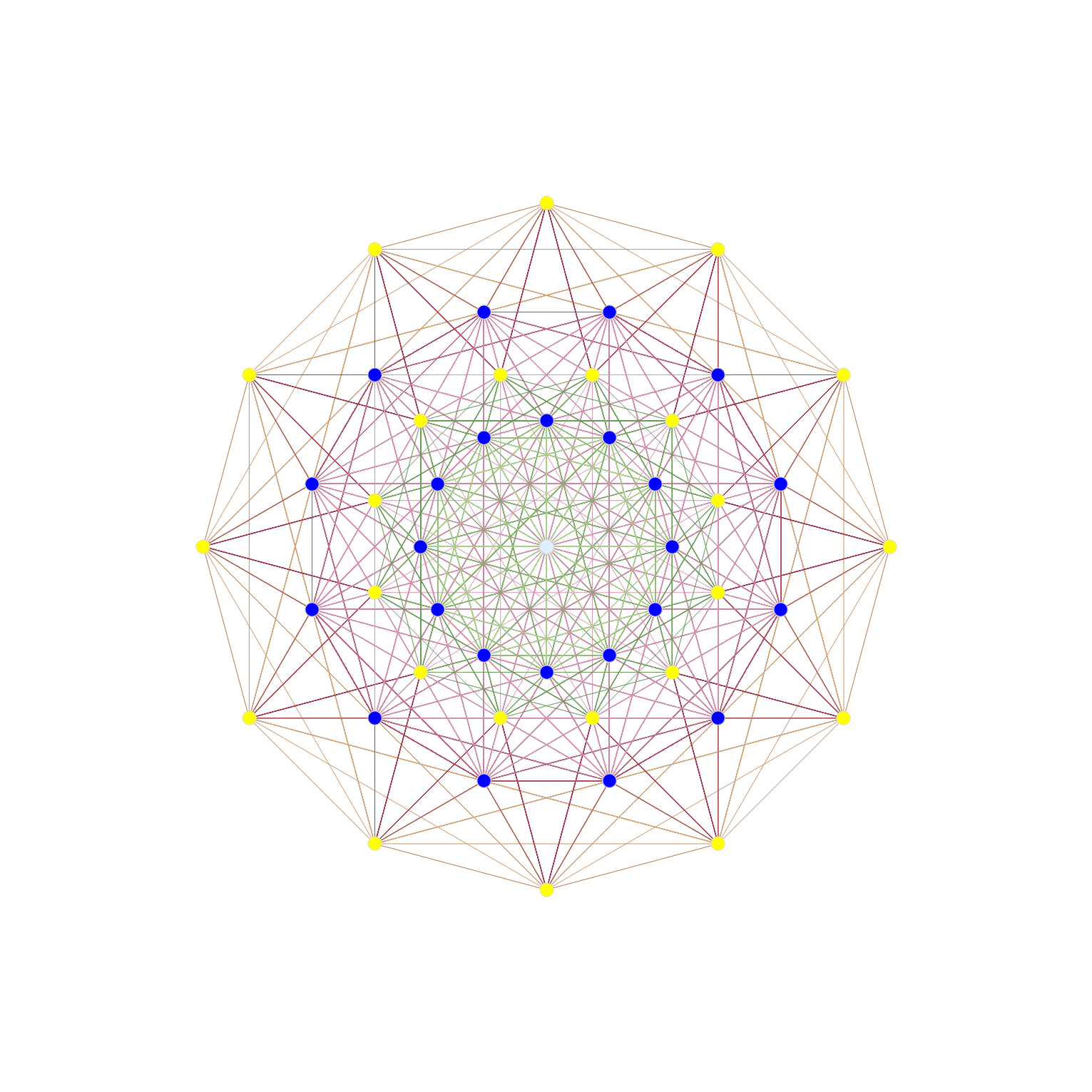The projection uses X Y basis vectors of:
X = {-Sqrt + 1, 0, 1, 1, 0, 0, 0, 0};
Y = {0, Sqrt – 1, -1, 1, 0, 0, 0, 0};

Resulting in vertex overlaps of:
24 Yellow with 1 overlap
24 Dark Blue each with 8 overlaps (192 vertices)
1 Light Blue with 24 overlaps (24 vertices)

After doing this for a few example symmetries, Tom took my idea of projecting higher dimensional objects to the 2D (and 3D) symmetries of lower dimensional subgroups – and ran with it in 2D – producing a ton of visualizations across WP. 🙂

It was one of those that was subsequently used that article from the 4_21 E8 WP page.

Here is a representation of E6 in the E6 Coxeter plane: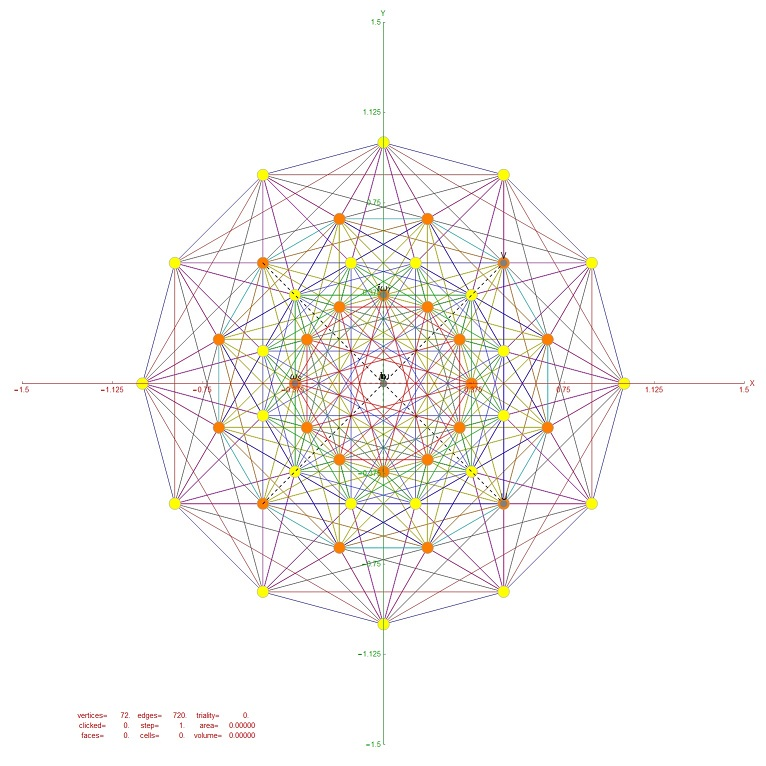Resulting in vertex overlaps of:
24 Yellow with 1 overlap
24 Orange each with 2 overlaps (48 vertices)

# My E8 F4 projection and so called "Sacred Geometry"

Please note, this is about the GEOMETRY used in what has come to be called “Sacred Geometry”. Upon further investigation, along with the Kabbalah’s “Tree of Life”, it seems even the Jewish “Star of David” (Solomon) is medieval and may not be sourced in authentic ancient Abrahamic theology.

As it stands, the so-called “sacred” origin of these, including the pentagon that shows up in the icosahedral/dodecahedral Platonic solids due to the Sqrt(5) Golden Ratio (Phi) relationship within my E8 to H4 folding, is likely medieval and mostly pagan. I am merely pointing out that E8 contains the geometry that many find “interesting” for whatever reason, and am not promoting the underlying cults that use them.

Video (Best in HD):

My E8 F4 projection w/blue triality edges and physics particle assignments:My E8 F4 projection with 6720 edge lines:Rectified E8 and F4 Triality (Original Work)

WikiMedia Commons source images:
Metatron’s Cube (User:Barfly2001)
Flower of Life (User:Life_of_Riley)
Tree of Life (User:AnonMoos)

# A new Lisi Arxiv paper along with a new E8 projection

For Lisi’s latest ideas on a “Lie Group Cosmology (LGC)” ToE, see http://arxiv.org/abs/1506.08073.
Be warned, it is over 40 heavy pages.

He also posted a new projection on FB.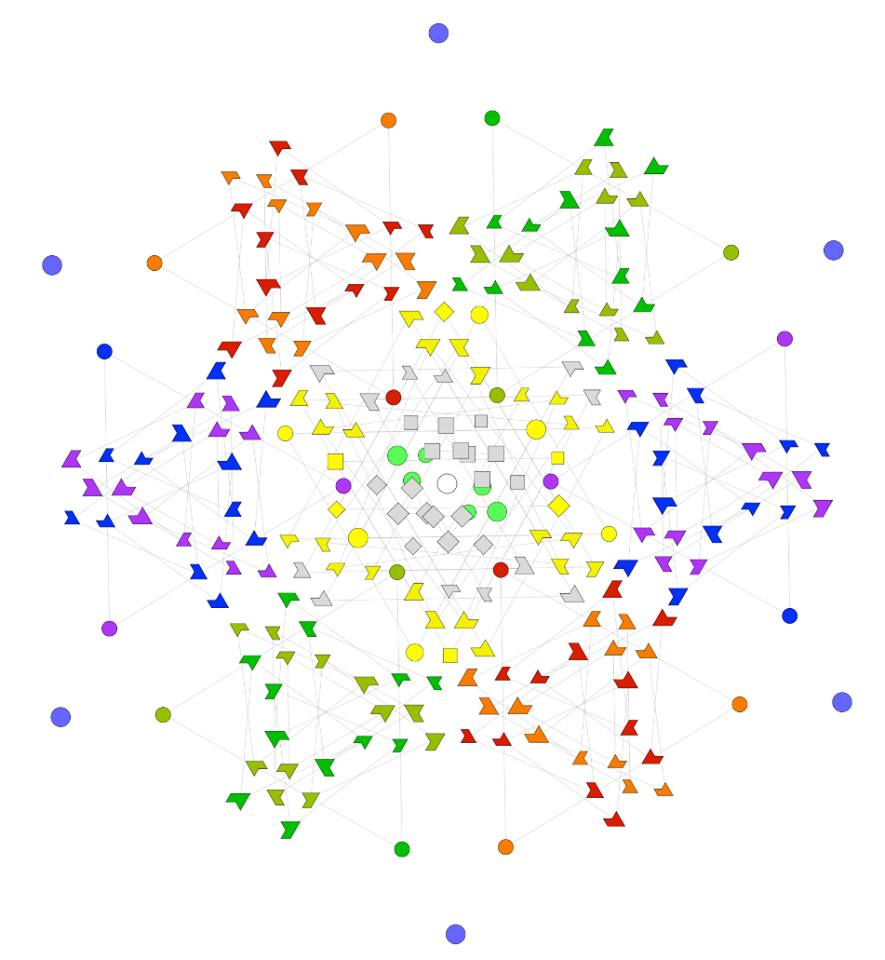He uses the (H)orizontal and (V)ertical projection vectors of:
H = {.12, -.02, .02, .08, .33, -.49, -.49, .63};
V = {-.04, .03, .13, .05, .20, .83, -.45, .19};

Here it is rendered with H and V reordered to match in VisibLie_E8 tool:
H/V order is {2, 3, 4, 7, 1, 6, 5, 8};
Note: the particle assignments in my VisibLie_E8 tool (as in the Interactive visualizations on this website) are not the same and have different triality rotation matrices.The pic below has a slightly altered projection that creates a bit more hexagonal symmetry and integer projections (without deviating more than .05 off his coordinates):
Note: vertex colors indicate the number of overlapping vertices.
This uses H/V projection vectors (reverse ordered back to his coordinate system).
Symbolic:
H={3 Sqrt/40, 0, 0, 3 Sqrt/40, 1/3, 1/2, 1/2, -2/3};
V={-3/40, 0, 3/20, 3/40, 3/20, -4/5, 1/2, -3/20}};
Numeric:
H={.13, 0., 0.,.13,.33,.5, .5,-.66`};
V={-.075, 0., .15, .075, .15,-.8, .5,-.15};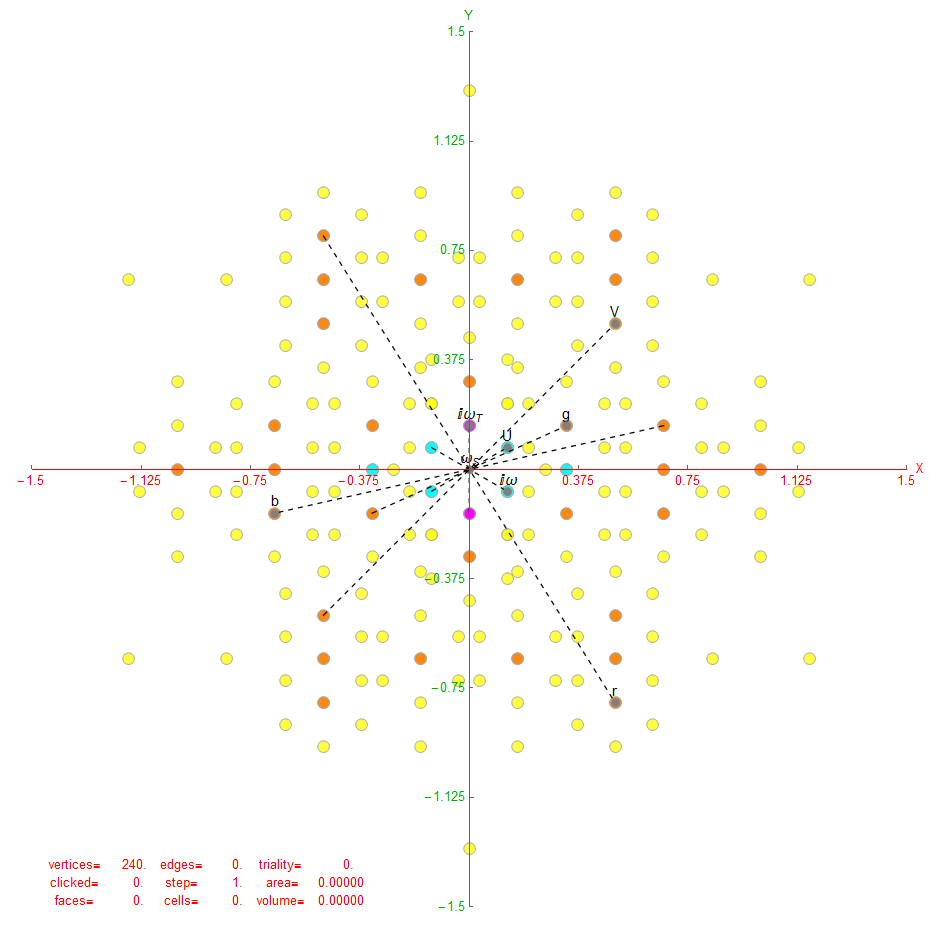Here is Lisi’s version with my coordinates:Here is a version of the full hexagonal (Star of David) triality representation using coordinates I found with the same process used above. Particle color, shape and size are based on the assigned particle quantum numbers (spin, color, generation). There are 86 trialities indicated by blue triangles.
Projection vectors (in my “physics” coordinate system) are:
H={2-4/Sqrt,0,0,-Sqrt[(2/3)]+Sqrt,0,0,Sqrt,0};
V={0,-2+4/Sqrt,Sqrt[2/3]-Sqrt,0,0,0,0,-Sqrt};See also a rectified version of this, where the particle overlaps show the inner beauty of E8!!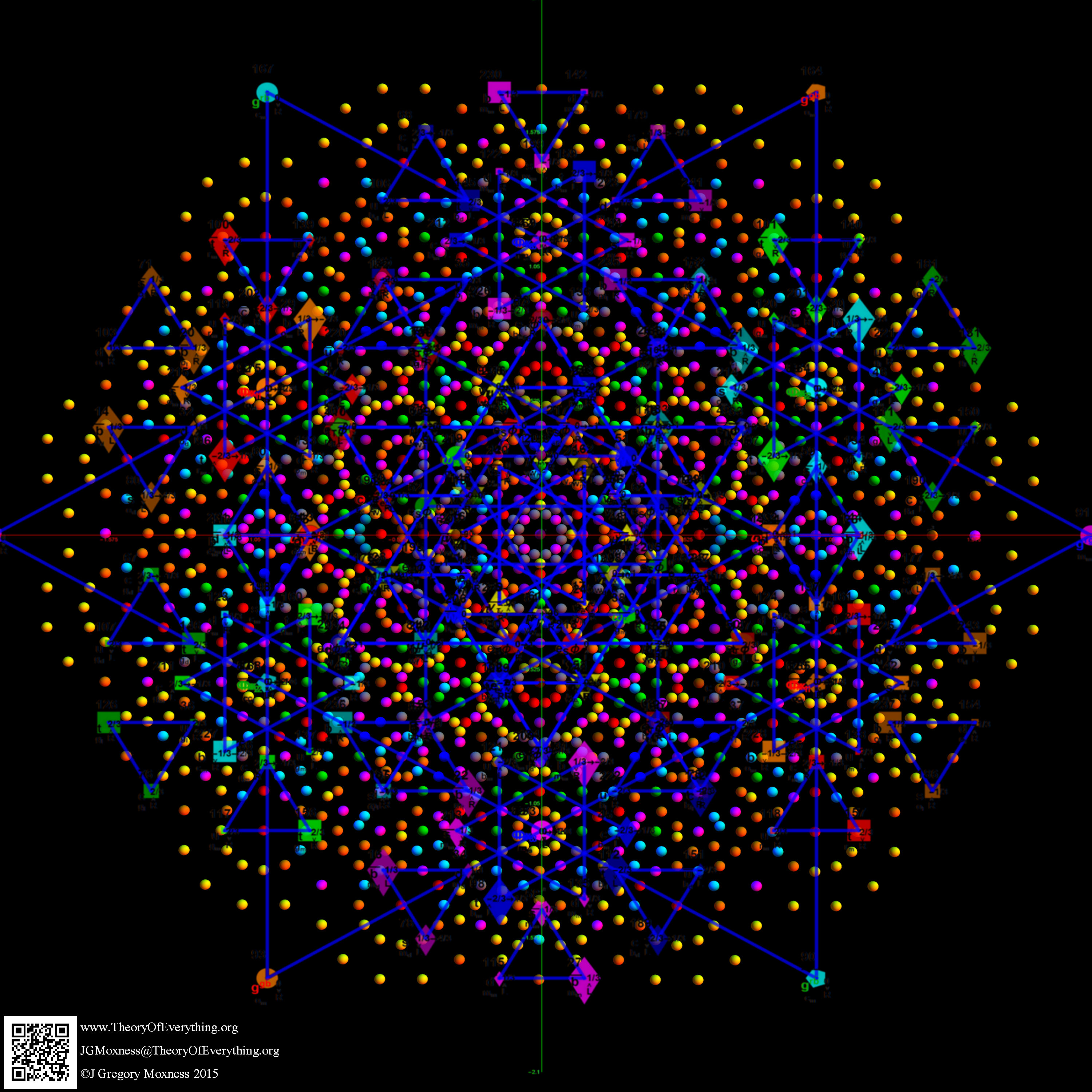The underlying quantum symmetry patterns within this coordinate system are: